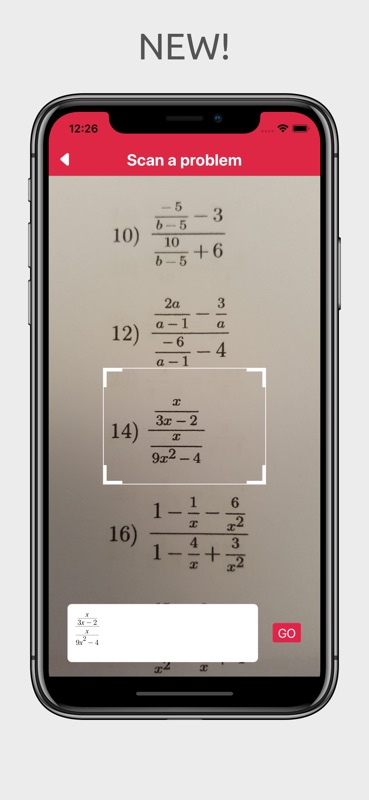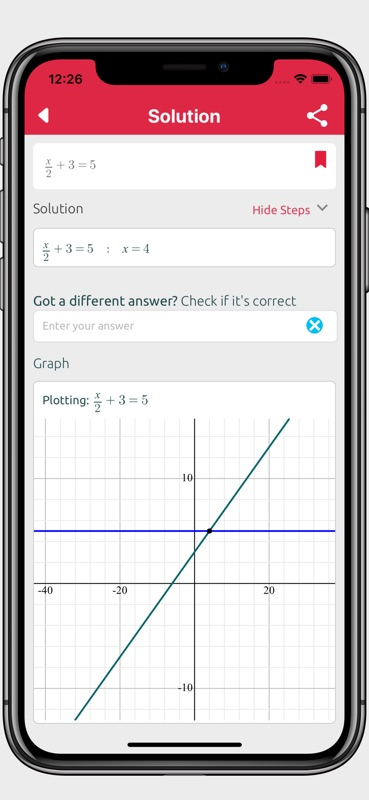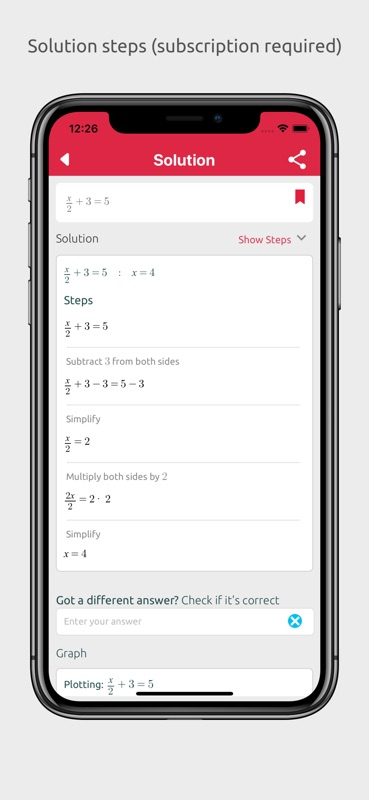## Symbolab Calculator

Math Solver with StepsYour private math tutor, solves any math problem with steps! Integrals, Derivatives, Equations, Limits and much more.
(Steps require a subscription.)

Symbolab Math Solver is composed of over a hundred of Symbolab most powerful calculators:
Integral Calculator
Derivative Calculator
Limit calculator
Equation Calculator
Inequality Calculator
Trigonometry Calculator
Matrix Calculator
Functions Calculator
Series Calculator
ODE Calculator
Laplace Transform Calculator

Symbolab Math Solver solves any math problem including Pre- Algebra, Algebra, Pre-Calculus, Calculus, Trigonometry, Functions, Matrix, Vectors, Geometry and Statistics.

Symbolab solves any problem for free. In order to view step-by-step solutions, you can subscribe weekly (\$0.99), monthly (\$2.49), or yearly (\$14.99).

• Payment will be charged to iTunes Account at confirmation of purchase
• Subscription automatically renews unless auto-renew is turned off at least 24-hours before the end of the current period
• Account will be charged for renewal within 24-hours prior to the end of the current period, and identify the cost of the renewal
• Subscriptions may be managed by the user and auto-renewal may be turned off by going to the user’s Account Settings after purchase

### In-App Purchases

#### Resources

• FREE All features with no ads
• FREE Universal Weekly Subscription
• FREE Universal Monthly Subscription
• FREE Universal Yearly Subscription
• FREE Integrals
• FREE Limits
• FREE Trigonometry Identities
• FREE Definite Integrals
• FREE Inequalities
• FREE Absoluts